Histogram Equalization

• ## 直方图均衡化的数学原理

万次阅读 多人点赞 2016-09-04 13:36:19
直方图均衡化的数学原理直方图均衡化处理的“中心思想”是把原始图像的灰度直方图从比较集中的某个灰度区间变成在全部灰度范围内的均匀分布。直方图均衡化就是对图像进行非线性拉伸，重新分配图像像素值，使一定灰度...
直方图均衡化的数学原理

直方图均衡化处理的“中心思想”是把原始图像的灰度直方图从比较集中的某个灰度区间变成在全部灰度范围内的均匀分布。直方图均衡化就是对图像进行非线性拉伸，重新分配图像像素值，使一定灰度范围内的像素数量大致相同。直方图均衡化就是把给定图像的直方图分布改变成“均匀”分布直方图分布【1】。有关直方图均衡化的深入介绍，可以参考作者的另一篇博客直方图匹配的数学原理（其中包含为什么要选用累积分布函数做映射函数?以及推导过程）。

下图为直方图均衡化的过程，体现了“均衡”的含义：（概率密度的均匀）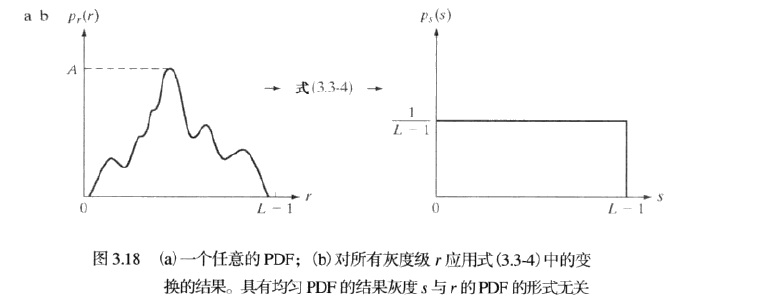网上可以查阅到关于直方图均衡化的各种解释、用法、程序、优缺点，这里只关注直方图均衡化的数学原理（可以参阅【1】）。

我们知道直方图变换其实是一种灰度变换，灰度变换的变换函数决定了输入随机变量与输出随机变量之间的关系，也就是两个随机变量的关系；一副图像是二维离散的数据，不利于使用数学的工具进行处理，在数字图像处理中，我们通常是采用连续的变量进行推导，最后在推广到离散的情况。

以下我们介绍：1.图像直方图的定义 2.直方图变换的理论基础（为什么介绍它？直方图变换是直方图均衡化的基础）3.概率知识-随机变量的函数分布 4.直方图均衡化的理论基础 5.离散形式的直方图均衡化。

一、图像直方图的定义

1.定义（1）：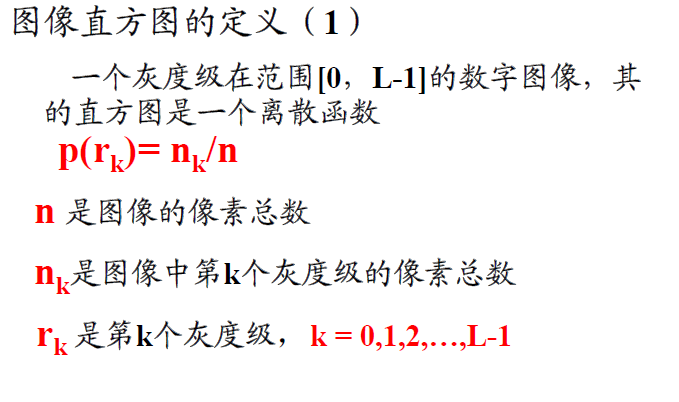2.定义（2）：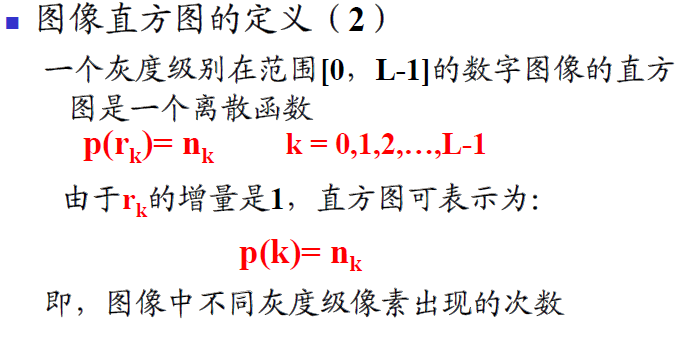3.比较：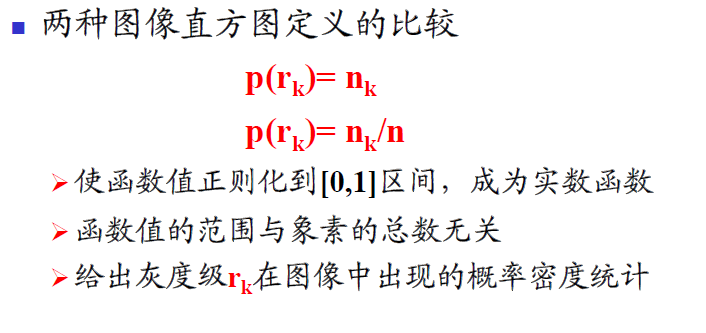二、直方图变换的理论基础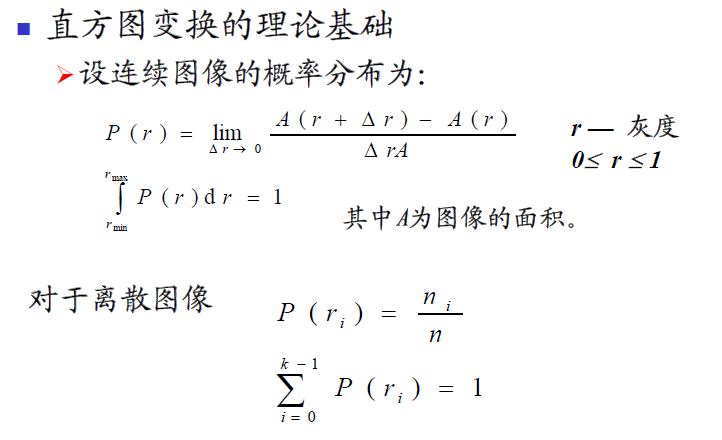注意：T（r）为单值单调增加（此为数字图像处理-冈萨雷斯-第二版中的表述），在第三版中有一些变化，具体的可以查阅此书的对应章节。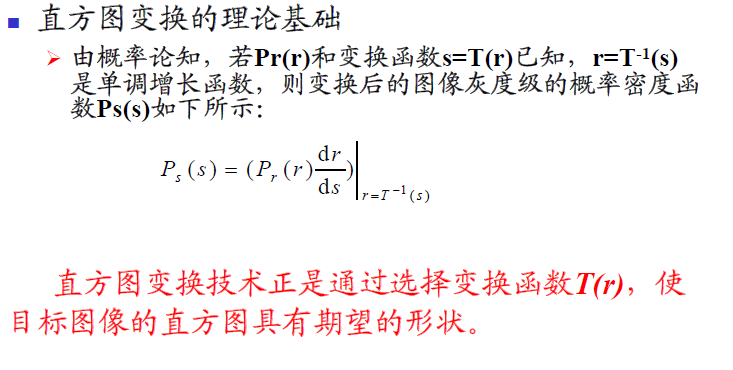三、概率论的知识-随机变量的函数的分布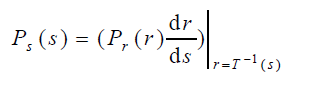这一块涉及了概率论的知识-随机变量的函数的分布：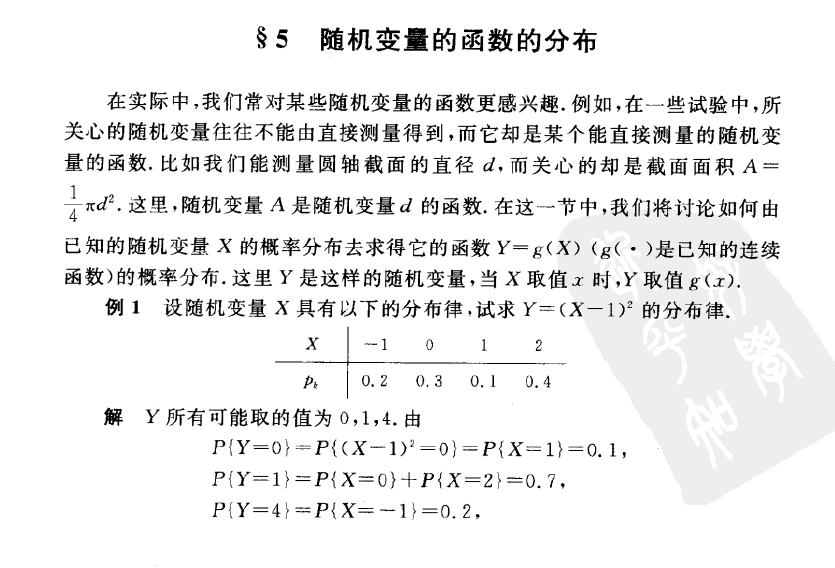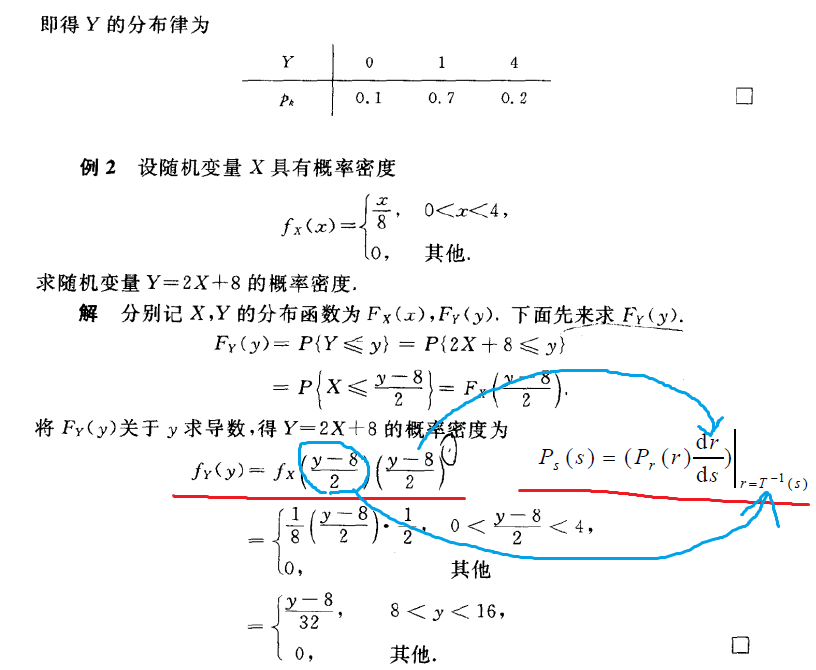四、直方图均衡化的理论基础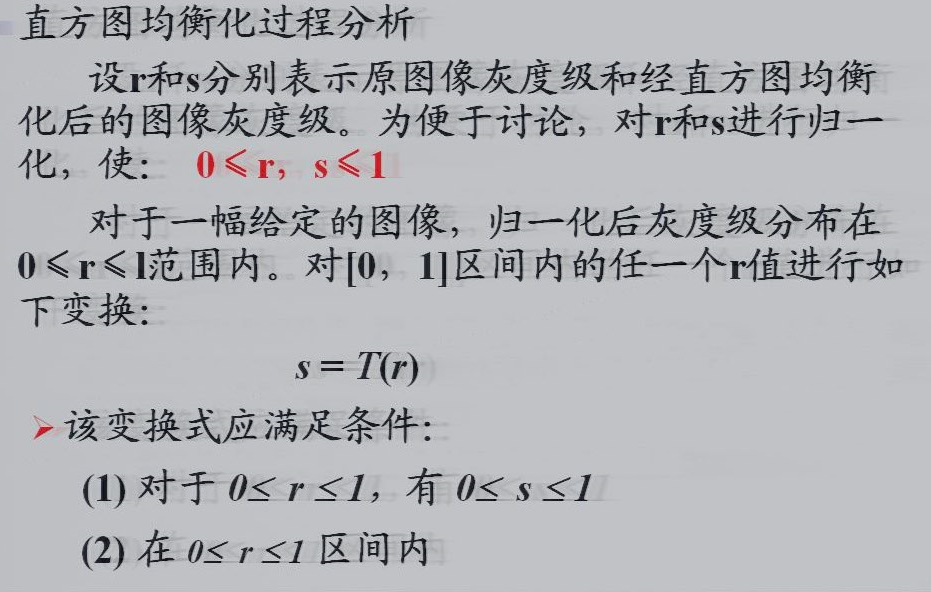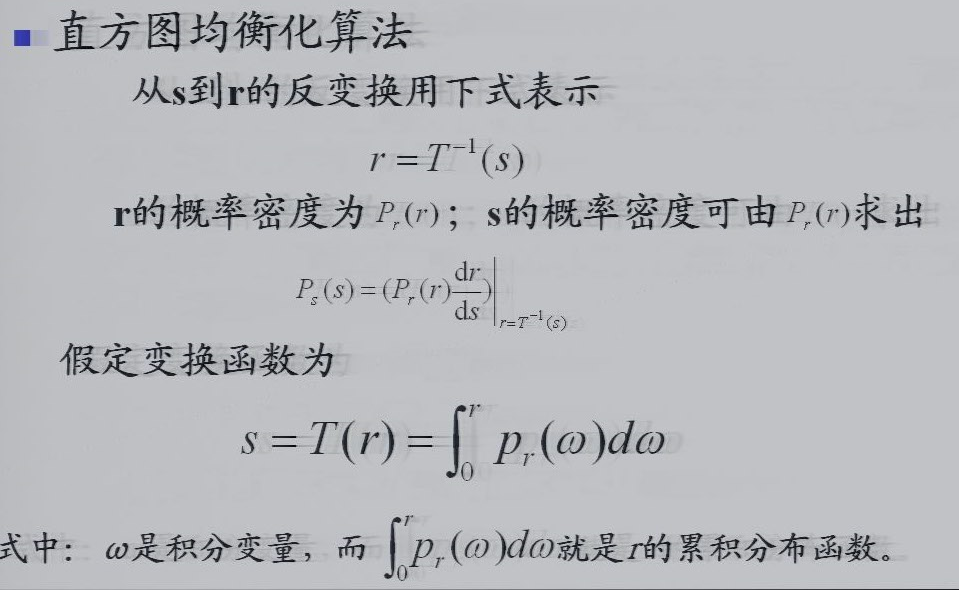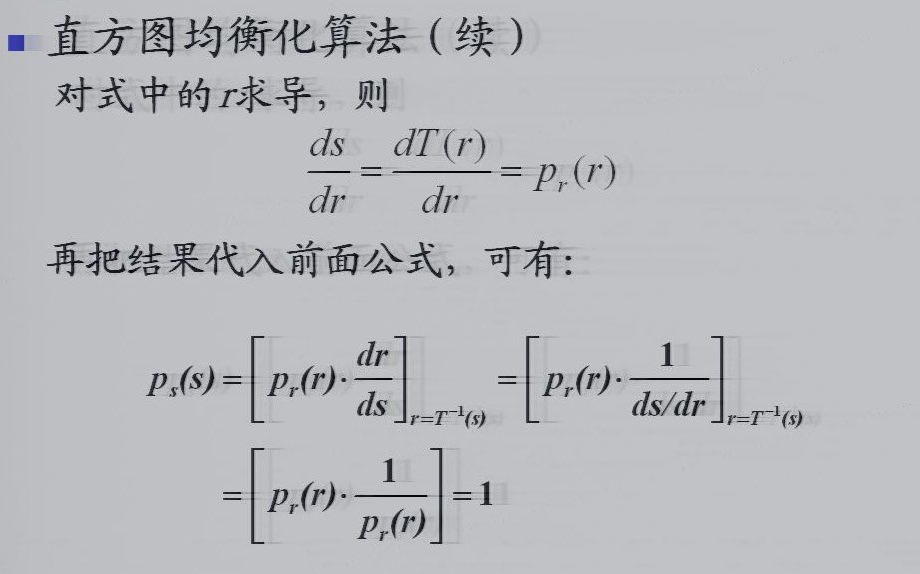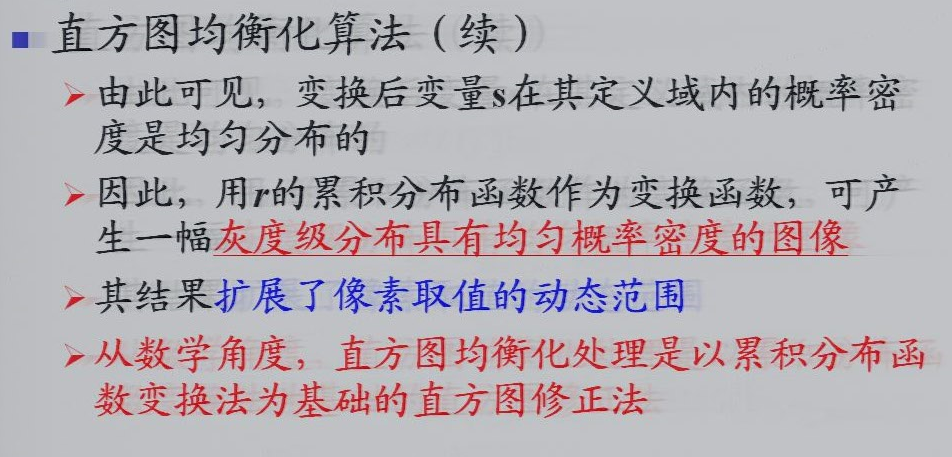五、离散形式的直方图均衡化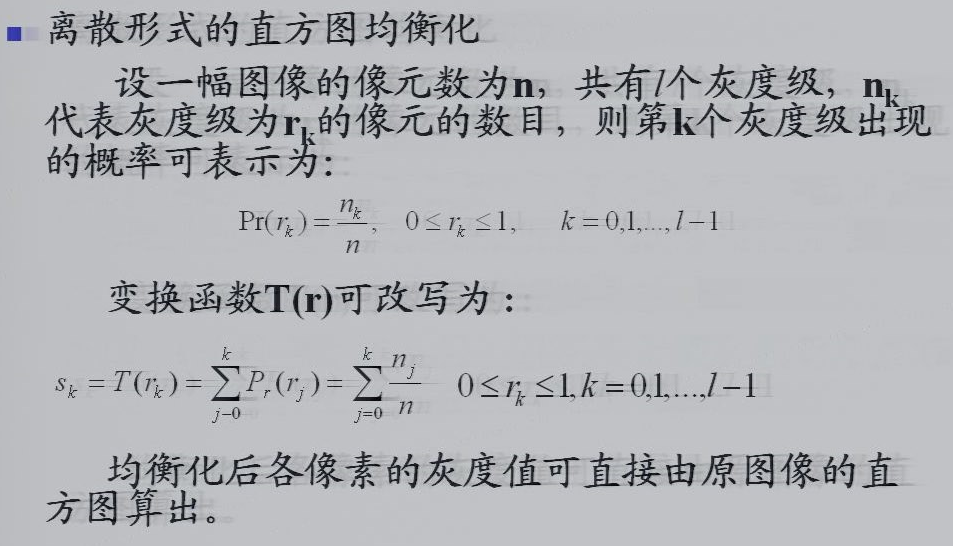六、例子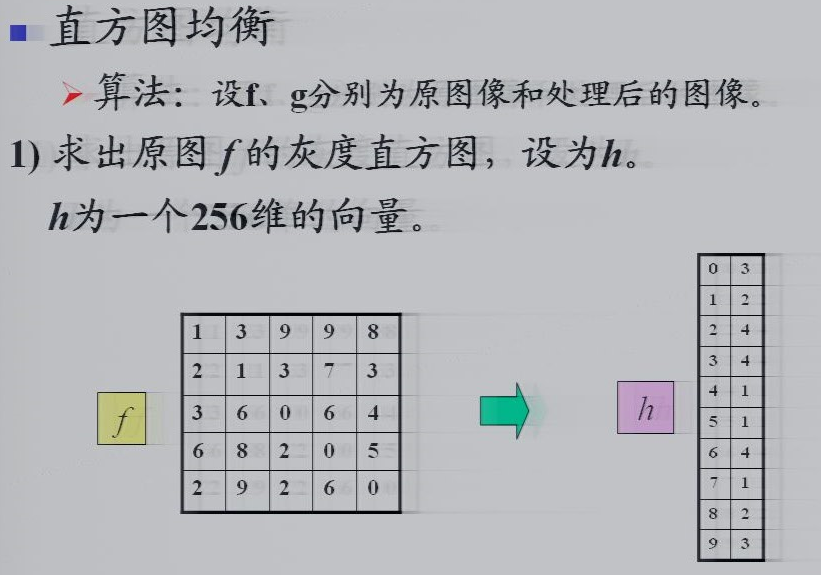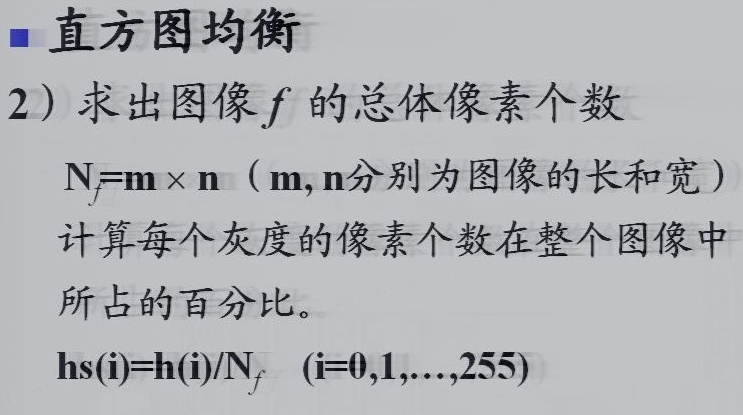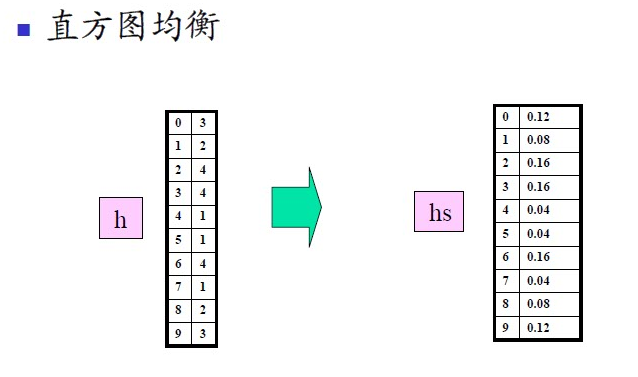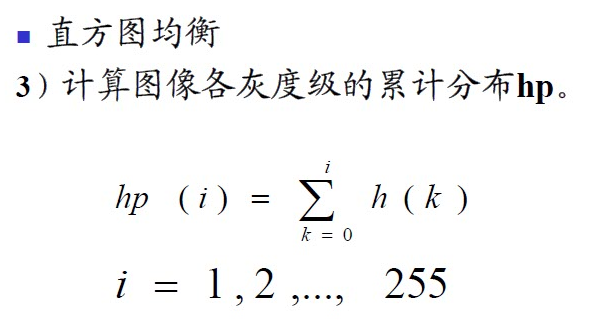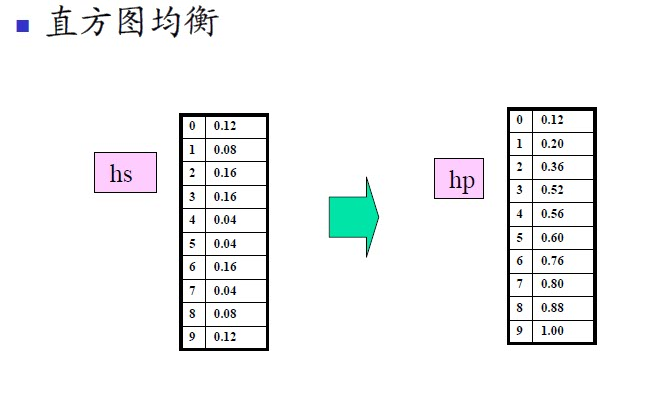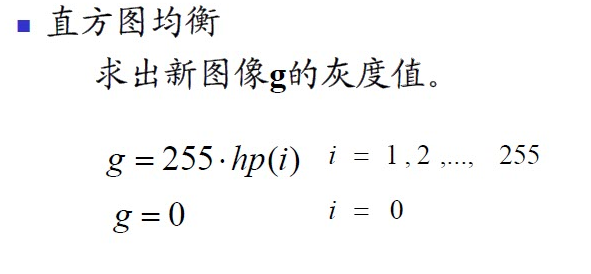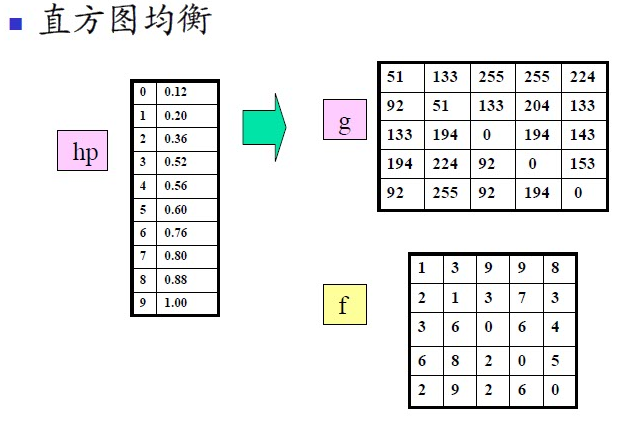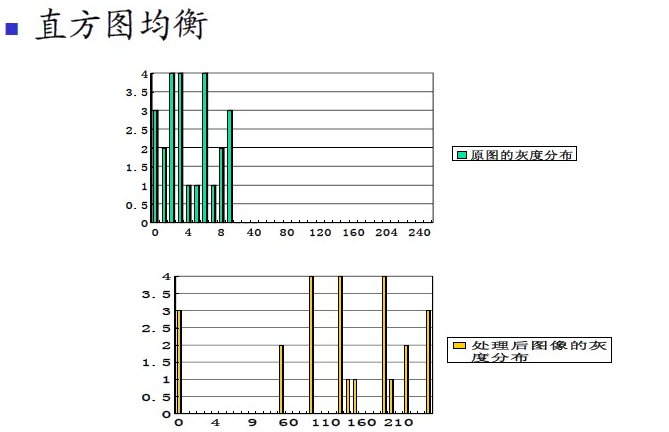参考资料：

【1】：【数字图像处理】直方图均衡化详解及编程实现
http://blog.csdn.net/xiajun07061225/article/details/6910129

参考书籍：

【2】：数字图像处理-冈萨雷斯-第二版

【3】：数字图像处理-冈萨雷斯-第三版

【4】：《概率论与数理统计》浙大版（第四版）


展开全文数学 图像处理
• ## 直方图均衡化算法原理与实现

万次阅读 多人点赞 2016-09-03 11:51:13
直方图均衡化算法原理与实现
工作后，对原来学习的一些基本图像处理算法有了一些新的认识，比如Canny 算法，直方图均衡化算法等，今天就来说说直方图均衡化算法。

直方图均衡化原理
我们知道提高图像对比度的变换函数$f(x)$需要满足一下条件：

$f(x)$在$0<=x<=L-1$上单调递增(不要求严格单调递增),其中L表示灰度级（L=256）
$f(x)$的范围是$[0,L-1]$

我们知道当图像直方图完全均匀分布的时候，此时图像的熵是最大的（随机变量每个值的概率都相同时，概率最大），图像对比度是最大的。所以，理想情况下，图像经过变换函数$f(x)$变换后，直方图能够均匀分布，此时对比度是最大的。
那问题来了？怎样的变换函数具有如此神奇的功能呢？P74中给出了答案。
在图像处理中，有一个重要的函数，能够满足上面的条件：
$y=f(x)=(L-1)\int_0^xp_x(t){\rm d}t$
英文原版中P145中的表述为：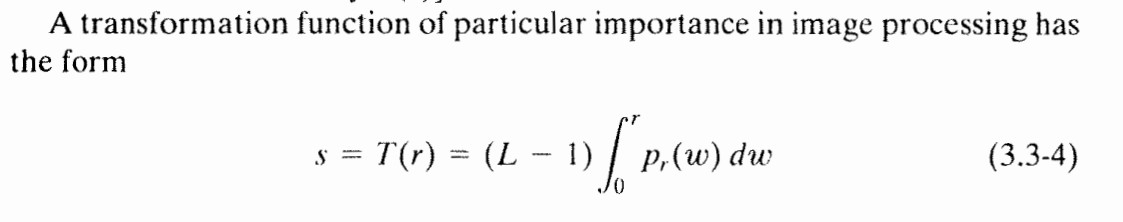其中$p_x(x)$表示概率密度函数，在离散的图像中，表示直方图的每个灰度级的概率（在图像中，灰度级就可以看成是一个随机变量，而直方图就是该随机变量的概率密度函数），由概率论的知识，我们可以知道，变换函数$f(x)$其实就是连续型随机变量x的分布函数，表示的是函数下方的面积。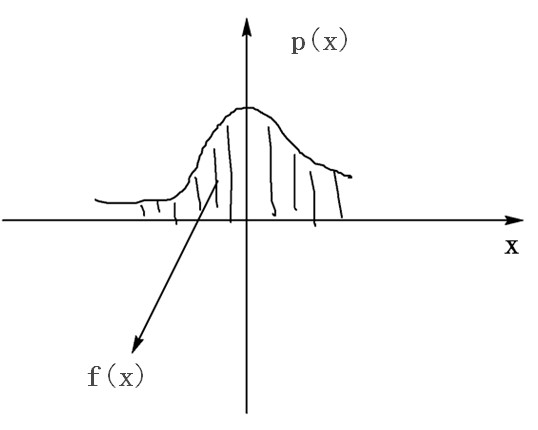分布函数的两个性质：1.单调不减  2.值域为[0,1]，我们可以知道f(x)满足条件1和2
有人可能会有这个疑问？图像是离散的，为什么可以用连续的来表示呢？从数学角度来看，离散是连续的一种特例（图像就是一个很好的例子）。
下面我们证明变换后的直方图是均匀的。
由概率论知识，变换后的概率密度：
$p_y(y)=p_x(x)|(f^{-1}(y))^\prime|$
由变上限函数求导法则可知$f(x)^\prime =(L-1)p_x(x)$
反函数的导数等于原函数导数的倒数，所以
$(f^{-1}(y))^\prime = {1 \over (L-1)p_x(x)}$
所以
$p_y(y)={1 \over L-1}$
看到了吧，变换后的概率密度函数是一个均匀分布，对于图像来说，就是每个灰度级概率都是相等的，达到了我们的目的。
下面我们需要将这个变换函数转换为图像中的表达，图像中，我们可以知道，可以使用求和代替积分，差分代替微分，所以上述的变换函数就是：
$y=f(x)=(L-1)\sum_0^{x_i}{h(x_i) \over w \times h}$
其中$h(x_i)$表示直方图中每个灰度级像素的个数， $w$和 $h$分别表示图像的宽和高。

直方图均衡化算法实现
根据上面的推导，算法实现如下：
//不支持OpenCV的ROI
void GetHistogram(const Mat &image, int *histogram)
{
memset(histogram, 0, 256 * sizeof(int));

//计算直方图
int pixelCount = image.cols*image.rows;
uchar *imageData = image.data;
for (int i = 0; i <= pixelCount - 1; ++i)
{
int gray = imageData[i];
histogram[gray]++;
}
}

void EqualizeHistogram(const Mat &srcImage, Mat &dstImage)
{
CV_Assert(srcImage.type() == CV_8UC1);
dstImage.create(srcImage.size(), srcImage.type());

// 计算直方图
int histogram;
GetHistogram(srcImage, histogram);

// 计算分布函数(也就是变换函数f(x))
int numberOfPixel = srcImage.rows*srcImage.cols;
int LUT;
LUT = 1.0*histogram / numberOfPixel*255;
int sum = histogram;
for (int i = 1; i <= 255; ++i)
{
sum += histogram[i];

LUT[i] = 1.0*sum / numberOfPixel * 255;
}

// 灰度变换
uchar *dataOfSrc = srcImage.data;
uchar *dataOfDst = dstImage.data;
for (int i = 0; i <= numberOfPixel - 1; ++i)
dataOfDst[i] = LUT[dataOfSrc[i]];
}


原图测试结果：从直方图均衡化算法中，可以看出，看似简单的几个算法步骤，背后蕴藏了很多数学理论知识，这大概就是数学的魅力吧。
完整工程见github项目：QQImageProcess_OpenCV
其中直方图均衡化算法的实现在 Src/ImageProcess/GrayTransformation.h中的EqualizeHistogram函数中。
同时自己也实现了一个不依赖于OpenCV的直方图均衡化算法(其实不依赖于任何第三方库)，见github项目：QQImageProcess，直方图均衡化算法的具体实现在Src/ImageProcess/GrayTransformation.h中的EqualizeHistogram()函数中，EqualizeHistogram()函数的使用示例见 Src/ImageProcessTest.h中的TestEqualizeHistogram()函数。

2016-9-3 10:41:39

参考文献

《数字图像处理》第3版，冈萨雷斯

非常感谢您的阅读，如果您觉得这篇文章对您有帮助，欢迎扫码进行赞赏。展开全文• ## 直方图均衡化

万次阅读 多人点赞 2018-11-29 20:54:03
图像的空域处理是一种重要的图像处理技术，这类方法直接以图像的像素操作为基础，主要分为灰度变换和空域滤波两大类，直方图均衡化（Histogram equalization）就是一种常用的灰度变换方法。 直方图 对于灰度级...
引言
图像的空域处理是一种重要的图像处理技术，这类方法直接以图像的像素操作为基础，主要分为灰度变换和空域滤波两大类，直方图均衡化（Histogram equalization）就是一种常用的灰度变换方法。
直方图
对于灰度级（intensity levels）范围为$[0, L-1]$的数字图像，其直方图可以表示为一个离散函数$h(r_k)=n_k$， 其中$r_k$是第k级灰度值（intensity value），$n_k$是图像中灰度值为$r_k$的像素个数，也就是说，图像的灰度直方图表征的是该图像的灰度分布。在实际应用中，通常对直方图进行归一化再进行后续处理，假设灰度图像的维数是M×N，MN表示图像的像素总数，则归一化直方图可以表示为
$p(r_k)=n_k/MN，k=0,1,…,L-1$也就是说，$p(r_k)$表示灰度级$r_k$在图像中出现概率的估计，归一化直方图所有分量之和等于1。
直方图均衡化
1. 问题描述
通常，暗图像直方图的分量集中在灰度较低的一端，而亮图像直方图分量偏向于灰度较高的一端，如下图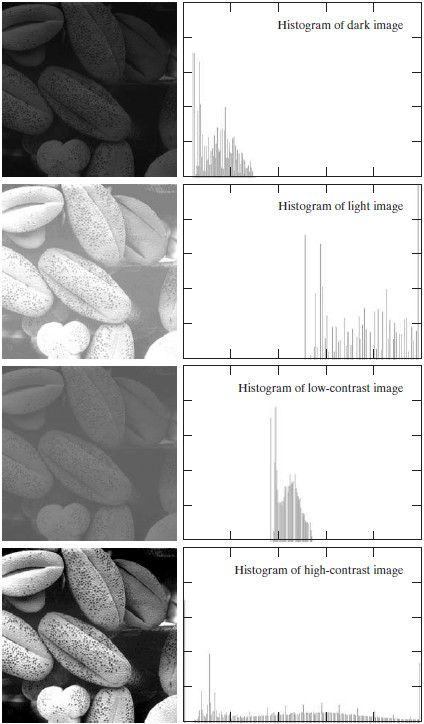从图中可以得到这样的结论：如果一幅图像的灰度直方图几乎覆盖了整个灰度的取值范围，并且除了个别灰度值的个数较为突出，整个灰度值分布近似于均匀分布，那么这幅图像就具有较大的灰度动态范围和较高的对比度，同时图像的细节更为丰富。已经证明，仅仅依靠输入图像的直方图信息，就可以得到一个变换函数，利用该变换函数可以将输入图像达到上述效果，该过程就是直方图均衡化。
下面从数学的角度理解直方图均衡化。假设待处理图像为灰度图像，$r$表示待处理图像的灰度，取值范围为$[0,L-1]$，则$r=0$表示黑色，$r=L-1$表示白色，直方图均衡化的过程对应于一个变换$T$：$s=T(r), 0 \leq r \leq L-1 i\tag{1}$也就是说，对于输入图像的某个灰度值$r$，可以通过变换$T$得到均衡化后的图像对应位置的灰度值$s$。其中变换$T$满足以下条件：
(a) $T(r)$在$[0,L-1]$上严格单调递增；
(b) 当$0 \leq r \leq L-1$时，$0 \leq T(r) \leq L-1$。
条件(a)中要求$T(r)$严格单调递增是为了保证输出灰度值与输入灰度值一一对应，同时像素灰度值之间的相对大小关系不变，这样可以避免反变换时出现问题；条件(b)保证了输出图像的灰度范围与输入图像相同。实际中处理的图像通常是整数灰度值，必须把所有结果四舍五入为最接近的整数值。因此，当严格单调条件不满足时，使用寻找最接近整数匹配的方法解决反变换不唯一的问题。
2. 均衡化推导
首先考虑连续形式，一幅灰度图像的灰度级可以看作区间 $[0,L-1]$ 内的随机变量，因此可用其概率密度函数(PDF)描述。假设 $p_r(r)$ 和 $p_s(s)$ 分别表示随机变量 $r$ 和 $s$ 的PDF，$p_r(r)$ 和变换 $T$ 已知，且 $T(r)$ 在定义域内连续可微，则变换后 $s$ 的PDF可由下式得到：$p_s(s)=p_r(r)|\frac{dr}{ds}|\tag{2}$

此处用到了概率论中有关随机变量函数分布的相关定理：设 $\xi$ 是连续型随机变量，其概率密度函数为 $p(x)$，又函数 $y=f(x)$
严格单调，其反函数 $h(y)$ 有连续导数，则 $\eta =f(\xi)$ 也是一个连续型随机变量，其概率密度函数为
$\psi(y)= \begin{cases} p[h(y)]\cdot|h'(y)|&,\alpha<y<\beta\\ 0&,其他 \end{cases}$ 其中 \begin{aligned} \alpha=min\{f(-\infty),f(+\infty)\}\\ \beta =max\{f(-\infty),f(+\infty)\} \end{aligned}

由此看到，输出图像灰度 $s$ 的PDF就由输入图像灰度 $r$ 的PDF和变换 $T$ 得到。

图像处理中一个重要的变换函数构造如下 $s=T(r)=(L-1)\int^r_0p_r(w){\rm d}x\tag{3}$
其中，$w$是形式积分变量，公式右边是随机变量$r$的累积分布函数(CDF)，可以看到，(3)式完全满足前述的条件(a)和(b)。

为寻找变换后随机变量 $s$ 的概率密度函数 $p_s(s)$，由(2)式得，
\begin{aligned} \frac{{\rm d}s}{{\rm d}r}&=\frac{{\rm d}T(r)}{{\rm d}r}\\ &=(L-1)\frac{\rm d}{{\rm d}r}\int^r_0p_r(w){\rm d}w=(L-1)p_r(r)\tag{4} \end{aligned}将(4)式代入(2)式得
\begin{aligned} p_s(s)&=p_r(r)|\frac{{\rm d}r}{{\rm d}s}|\\ &=p_r(r)\frac{1}{(L-1)p_r(r)}=\frac{1}{L-1}, 0\le s\le L-1 \tag{5} \end{aligned}由(5)式可知，$p_s(s)$ 为均匀分布，也就是说，输入图像的PDF经过(3)式中的变换 $T$ 后得到的随机变量 $s$ 服从均匀分布。
结论：图像均衡化变换 $T(r)$ 取决于 $p_r(r)$， 但得到的 $p_s(s)$ 始终是均匀的，与 $p_r(r)$ 的形式无关。
对于离散形式，其推导过程与连续形式相似，用概率直方图和求和运算分别代替概率密度函数和积分运算，可得(3)式的离散形式
\begin{aligned} s_k&=T(r_k)\\ &=(L-1)\sum ^k _{j=0}p_r(r_j)=\frac{L-1}{MN}\sum ^k_{j=0}n_j,k=0,1,2,\cdots,L-1\tag{6} \end{aligned}其中，$MN$为图像像素总数，$n_k$ 是灰度为 $r_k$ 的像素个数，$L$ 是图像可能的灰度级数量(例如对于8比特图像 $L=256$)。通过(6)式，输出图像中像素的灰度值可由输入图像中像素灰度 $r_k$ 映射为 $s_k$ 后得到。(6) 式中的变换（映射）$T(r_k)$ 称为直方图均衡或直方图线性变换。
3. 例子
假设一幅大小为 $64\times64$ 像素的3比特图像 $(L=8)$ 的灰度分布如表1所示，其中灰度级是范围 $[0,L-1]=[0,7]$ 中的整数。

$r_k$
$n_k$
$p_k(r_k)=n_k/MN$

$r_0=0$
790
0.19

$r_1=1$
1023
0.25

$r_2=2$
850
0.21

$r_3=3$
656
0.16

$r_4=4$
329
0.08

$r_5=5$
245
0.06

$r_6=6$
122
0.03

$r_7=7$
81
0.02

则直方图均衡化的值可由 (6) 式计算得到，例如
\begin{aligned} s_0&=T(r_0)=7\sum^0_{j=0}p_r(r_j)\\ &=7p_r(r_0)=7\times 0.19=1.33 \end{aligned}同理，\begin{aligned} s_1&=T(r_1)=7\sum^1_{j=0}p_r(r_j)\\ &=7p_r(r_0)+7p_r(r_1)=7\times 0.19+7\times 0.25=3.08 \end{aligned}以此类推计算求得所有 $s_k$ 值并将其近似为最接近的整数，结果如下：
\begin{aligned} &s_0=1.33\rightarrow 1,&&s_4=6.23\rightarrow 6\\ &s_1=3.08\rightarrow 3, &&s_5=6.65\rightarrow 7\\ &s_2=4.55\rightarrow 5, &&s_6=6.86\rightarrow 7\\ &s_3=5.67\rightarrow 6, &&s_7=7.00\rightarrow 7 \end{aligned}可以看到，均衡化后的图像只有5个灰度级，$r_0=0$ 被映射为 $s_0=1$，在均衡化后的图像中有790个像素取该值（见表1），有1023个像素取 $s_1=3$，有850个像素取 $s_2=5$，有656+329=985个像素取6，因为 $r_3$ 和 $r_4$ 都被映射为6这个值，同理，有245+122+81=448个像素取7。
因为直方图是PDF的近似，并且处理后不产生新的灰度级，所以在实际的直方图均衡化很少能够得到完全平坦的直方图。在离散情况下，通常不能证明离散的直方图均衡化能得到均匀的直方图。尽管如此，均衡化有助于图像直方图的延展，均衡化后图像的灰度级范围更宽，有效地增强了图像的对比度。同时，上述方法完全是“自动”的，仅利用输入图像的直方图信息，无需更多的参数。
Reference
Gonzalez, Rafael C., and Richard E. Woods. “Digital image processing.”


展开全文图像处理
• ## matlab实现直方图均衡化

万次阅读 多人点赞 2018-04-16 15:34:34
直方图均衡化是一种利用灰度变换自动调节图像对比度质量的方法，基本思想是通过灰度级的概率密度函数求出灰度变换函数，它是一种以累计分布函数变换法为基础的直方图修正法。直方图均衡化处理的步骤如下：1. 求出...
直方图均衡化是一种利用灰度变换自动调节图像对比度质量的方法，基本思想是通过灰度级的概率密度函数求出灰度变换函数，它是一种以累计分布函数变换法为基础的直方图修正法。直方图均衡化处理的步骤如下：1. 求出给定待处理图像的直方图2. 利用累计分布函数对原图像的统计直方图做变换，得到新的图像灰度。3. 进行近似处理，将新灰度代替旧灰度，同时将灰度值相等或相近的每个灰度直方图合并在一起MATLAB实现直方图均衡化处理的函数是：J=histeq（I，n）：I为输入的原图像，J为直方图均衡化后得到的图像，n为均衡化后的灰度级数，默认值为64直方图均衡化操作是对图像直方图进行处理，使得处理后的直方图为平坦形状。函数histeq（）不仅能够对灰度图像进行直方图均衡化，还可以对索引图像进行直方图均衡化。函数histeq（）对图像进行直方图均衡化处理实例：close all;clear all;clc;
%函数histeq（）进行直方图均衡化处理
J=histeq(I);  %直方图均衡化
figure,
subplot(121),imshow(uint8(I));
title('原图')
subplot(122),imshow(uint8(J));
title('均衡化后')
figure,
subplot(121),imhist(I,64);
title('原图像直方图');
subplot(122),imhist(J,64);
title('均衡化后的直方图');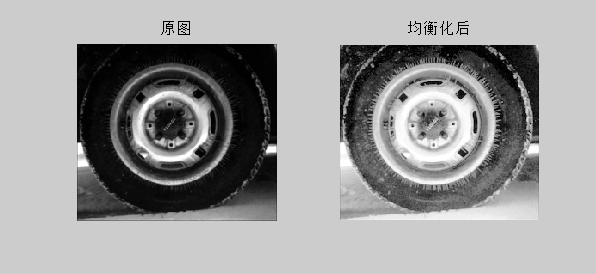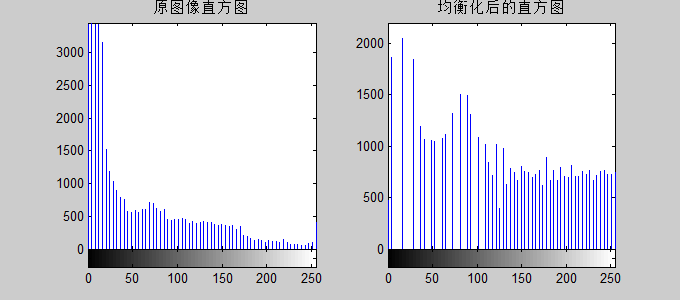------坚持就是胜利------
展开全文MATLAB histeq
• 直方图均衡化的英文名称是Histogram Equalization. 　图像对比度增强的方法可以分成两类:一类是直接对比度增强方法;另一类是间接对比度增强方法。直方图拉伸和直方图均衡化是两种最常见的间接对比度增强方法。直方...图像处理 matlab function
• ## 图像直方图与直方图均衡化

万次阅读 多人点赞 2016-01-29 12:47:11
图像直方图与直方图均衡化图像直方图以及灰度与彩色图像的直方图均衡化图像直方图：概述：图像的直方图用来表征该图像像素值的分布情况。用一定数目的小区间(bin)来指定表征像素值的范围,每个小区间会得到落入该小区...计算机视觉 python 图像直方图 线性插值
• ## 直方图均衡化原理

千次阅读 2018-08-13 18:18:48
直方图均衡化的作用是图像增强。 有两个问题比较难懂，一是为什么要选用累积分布函数，二是为什么使用累积分布函数处理后像素值会均匀分布。 第一个问题。均衡化过程中，必须要保证两个条件：①像素无论怎么映图像处理 opencv
• 图像处理开发资料、图像处理开发需求、图像处理接私活挣零花钱，可以搜索公众号"qxsf321"，并关注！ ...提问：图像直方图均衡化有啥效果？ 答：看了下面的两幅截图你就知道了： ...
•opencv
• 目录直方图均衡化步骤原理快速计算 直方图均衡化 直方图均衡化是将一幅图像的灰度直方图变平，使变换后的图像中每个灰度值的分布概率都相同。并且可以增强图像的对比度。 灰度值的分布概率都相同 这句话的理解，并不...opencv 计算机视觉 算法
• 直方图均衡化是基于灰度直方图的图像增强的一种方法，还有另外一种方法是直方图规定化。 均衡化的目的是将原始图像的直方图变为均衡分布的的形式，将一非均匀灰度概率密度分布图像，通过寻求某种灰度变换，变成一幅...图像处理 图像增强
• 首先感谢以下三位的博文帮助我理解了直方图均衡化（1）天涯路清晨 https://www.cnblogs.com/tianyalu/p/5687782.html（2）zrongh http://blog.csdn.net/zrongh/article/details/7302816（3）飞夺泸定桥 ...图像处理
• 直方图均衡化(Histogram Equalization)是一种增强图像对比度(Image Contrast)的方法，其主要思想是将一副图像的直方图分布变成近似均匀分布，从而增强图像的对比度。直方图均衡化虽然只是数字图像处理(Digital Image...
•python 图片
• DATE: 2019-7-8【Tags：直方图均衡化】 1、参考 2、直方图和直方图均衡化原理 3、算法实现图像处理
• DATE: 2019-11-1 1、参考 2、局部直方图均衡化原理 2.1、AHE 2.2、CLAHE 2.3、CLHE
• 直方图均衡化、直方图变换、对比度自适应直方图均衡化
•  直方图均衡化是通过拉伸像素强度分布范围来增强图像对比度的一种方法.  说得更清楚一些, 以上面的直方图为例, 你可以看到像素主要集中在中间的一些强度值上. 直方图均衡化要做的就是 拉伸 这个范围. 见下面...
• //对图像进行灰度线性bianh BOOL CDibImage::LinerTrans(LPSTR lpDIBBits, LONG lWidth, LONG lHeight,   FLOAT fA, FLOAT fB) { unsigned char* lpSrc; // 指向源图像的指针 LONG i; ...c++ 图像处理...